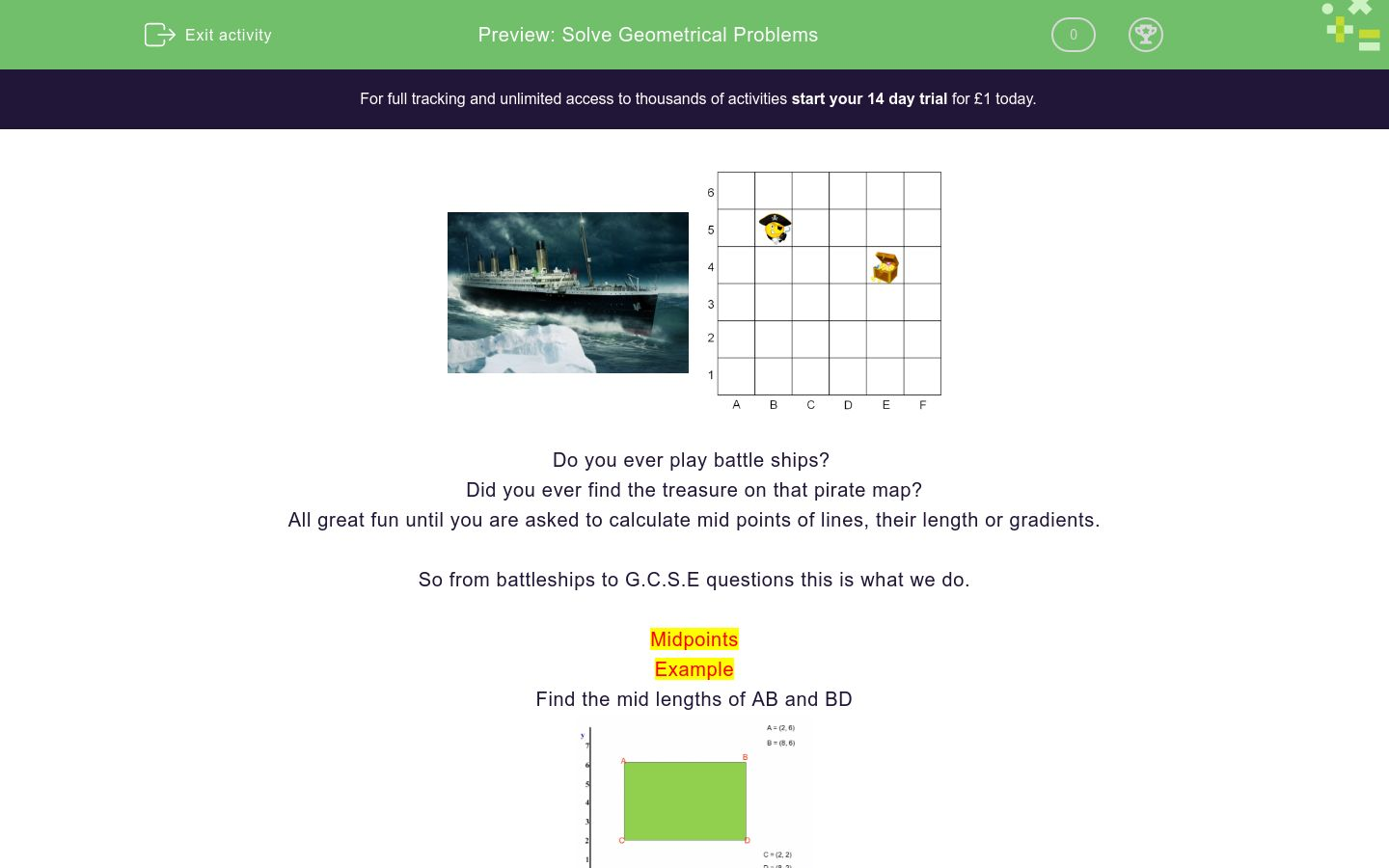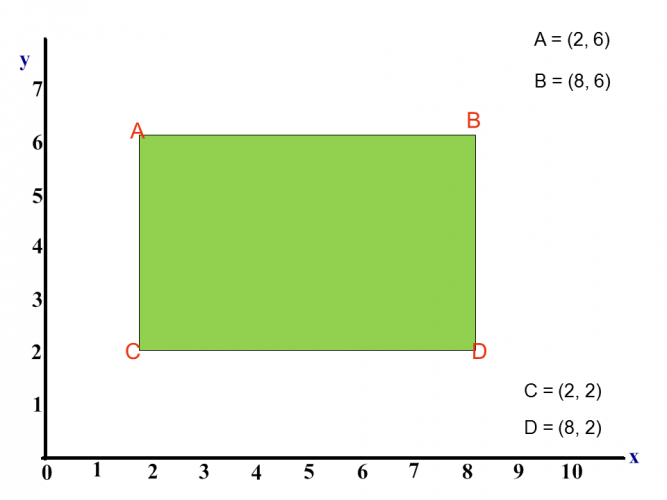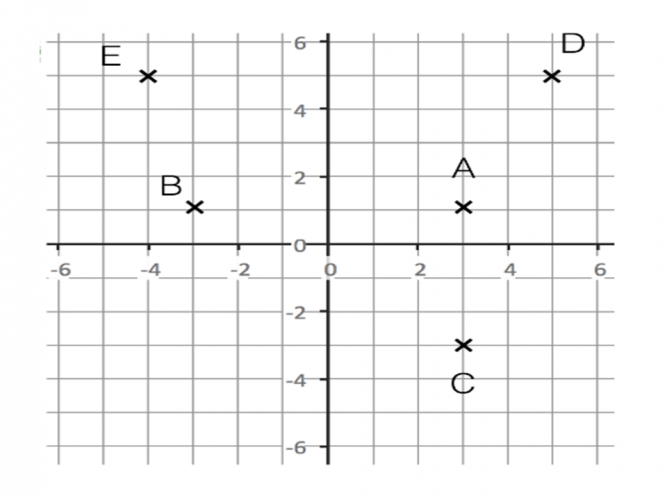# Solve Geometrical Problems

In this worksheet, students will use coordinates to find midpoints, line lengths and gradients of lines.Key stage:  KS 4

GCSE Subjects:   Maths

GCSE Boards:   OCR, AQA, Eduqas, Pearson Edexcel

Curriculum topic:   Basic Geometry, Geometry and Measures

Curriculum subtopic:   Conventions, Notations and Terms, Properties and Constructions

Difficulty level:### QUESTION 1 of 10Do you ever play battle ships?

Did you ever find the treasure on that pirate map?

All great fun until you are asked to calculate mid points of lines, their length or gradients.

So from battleships to G.C.S.E questions this is what we do.

Midpoints

Example

Find the mid lengths of AB and BDMid point of line AB

Take both x co-ordinates and add 8 + 2 = 10. Now divide by 2 = 5

Take both y co-ordinates and add 6 + 6 = 12. Now divide by 2 = 6

Mid point = (5,6)

Mid point of line BD

Take both x co-ordinates and add 8 + 8 = 16. Now divide by 2 = 8

Take both y co-ordinates and add 6 + 2 = 8. Now divide by 2 = 4

Mid point = (8,4)

You don't actually need a diagram you can do this without.

Find the mid point of AB.  A = (2,7)   B =  (6,5)

2 + 6 = 8 ÷ 2 = 4

7 + 5 = 12 ÷ 2 = 6

Mid point is (4,6)

Line Lengths1. Write out the co-ordinates P = (5,3) and Q = (1, -2).

On the diagram you can see that these co-ordinates make a right angled triangle.

2.  Find the difference between the x co-ordinates.

3. The difference between x is 4 and the difference between y is 5.

4. Now apply Pythagoras's Theorem 4² + 5² = √ 41. 6.4 units correct to 1 decimal place.

Gradients are written in the form y = mx + c.  The gradient is represented by the letter m.Draw a right angle anywhere on the line. Count the units.

Gradient is rise ÷ run (vertical ÷ horizontal)

4 ÷ 5 = 1.5 units

Like the others you can find a gradient without any diagram

Find the gradient of the line with coordinate points A = (4,9) B = (6,13)

Here is the formulaIt just means take the second y co-ordinate and subtract the first y co-ordinate from it.

Do the same for the x coordinates and then divide.

13 - 9 = 4

6 - 4 = 2

4 &divide;2 = 2

Gradient of the line is 2.Lets get coordinated to find that treasureThe coordinates of this line are (1,4) and (5,8)

What are the coordinates of the midpoint of this line?

(4,2)

(6,3)

(3,6)

(2,4)(4,2)

(6,3)

(3,6)

(2,4)What is the mid point of the line AB?

A = (3,9) B= (6,11)

Match the mid points to their coordinates.

## Column B

(2,5)(6,7)
(1, 7.5)
(7,2)(4,1)
(-4,5)
(-3,4)(-5,6)
(4,6)
(-4,-9)(-2,17)
(5.5,1.5)
(-2,7)(4,8)
(-3,1)
(-7,3)(1,5)
(-3,4)(100,0)

(0,100)

(60,0)

(0,60)Find the line length of AD.Find the line length of BC

 8.6 7.9 11.0 12.3 The length of BC is

Match up the line lengths with the coordinates.

## Column B

(3,9)(6,11)
6.1
(7,2)(4,1)
3.2
(-3,4)(-5,6)
2.8
(-2,7)(4,8)
4.7What is the gradient of the line BD.

 0.5 1 1.5 2 Gradient of the line BD isWhat is the gradient of line AD. A = (1,4) and D = (-2.-4)

• Question 1The coordinates of this line are (1,4) and (5,8)

What are the coordinates of the midpoint of this line?

(3,6)
EDDIE SAYS
Good news is that this is just adding up and dividing. The hard bit is remembering how to find the mid point in the first place. 1 + 5 = 6 ÷ 2 = 3 8 + 4 = 12÷ 2 = 6 Mid point is (3,6)
• Question 2EDDIE SAYS
120 + 0 = 120 ÷2; = 60 0 + 120 = 120 ÷2 = 60 Mid point (60,60)
• Question 3What is the mid point of the line AB?

A = (3,9) B= (6,11)

(4.5,10)
EDDIE SAYS
Who needs a diagram? If it is easier for you to visualise things always sketch out what you have been told. Once you know the coordinates just add and divide by 2. 3 = 6 = 9 ÷ 2 = 4.5 9 + 11 = 20 ÷2 = 10 (4.5, 10)
• Question 4

Match the mid points to their coordinates.

## Column B

(2,5)(6,7)
(4,6)
(7,2)(4,1)
(5.5,1.5)
(-3,4)(-5,6)
(-4,5)
(-4,-9)(-2,17)
(-3,4)
(-2,7)(4,8)
(1, 7.5)
(-7,3)(1,5)
(-3,1)
EDDIE SAYS
I hope you are getting used to doing these without a diagram. Add and Divide and you will glow with pride. 6 in a row and its go, go ,go
• Question 5(0,100)
(60,0)
EDDIE SAYS
And just when you though things were going well. We know that M is going to B (0,?) as it is directly on the Y axis. The y co-ordinate of the midpoint is 50. Instead of dividing by 2 we multiply by 2. Likewise on the x axis we know that the coordinate with be (?,0) The x coordinate of the mid point is 30, so double up. (60,0) Remember you can always reverse the process of doing things.
• Question 6Find the line length of AD.

8.5
EDDIE SAYS
This is where Pythagoras\' comes to join us. This time find the difference between the x coordinates and the y coordinates. A = (1,4) and D = (-2,-4) Difference between the x coordinates is 3 and the y coordinates 8. (if you have a diagram you can count the squares) Now we let Pythagoras\' do his stuff. 3² + 8² = √73 = 8.5 units correct to 1 decimal place.
• Question 7Find the line length of BC

 8.6 7.9 11.0 12.3 The length of BC is
EDDIE SAYS
B = (6,2) and D = (-5,1) Difference between the x coordinates is 11 and the y coordinates 1. And we are ready to go. 11² + 1² = √122 = 11.0 units correct to 1 decimal place.
• Question 8

Match up the line lengths with the coordinates.

## Column B

(3,9)(6,11)
4.7
(7,2)(4,1)
3.2
(-3,4)(-5,6)
2.8
(-2,7)(4,8)
6.1
EDDIE SAYS
Find the difference and apply Pythagoras. Where would we be without him. He pops up everywhere.
• Question 9What is the gradient of the line BD.

 0.5 1 1.5 2 Gradient of the line BD is
EDDIE SAYS
Here you need to take care to get your division the correct way round. Gradient is found like this. Rise ÷ Run (vertical ÷ horizontal. The units across (horizontal) 8 and the vertical units 4 (vertical) 4 ÷ 8 = - 0.5 .
• Question 10What is the gradient of line AD. A = (1,4) and D = (-2.-4)

2.7
EDDIE SAYS
Mid points, line lengths and gradients. You can find all of these without a diagram. y2 - y1 ÷ x2 - x1 4 - -4 = 8 and 1 - -2 = 3 8 ÷ 3 = 2.666 (2.7 correct to 1 decimal place) I hope these are now as easy to you as a game of battleships.
---- OR ----

Sign up for a £1 trial so you can track and measure your child's progress on this activity.

### What is EdPlace?

We're your National Curriculum aligned online education content provider helping each child succeed in English, maths and science from year 1 to GCSE. With an EdPlace account you’ll be able to track and measure progress, helping each child achieve their best. We build confidence and attainment by personalising each child’s learning at a level that suits them.

Get started